Next: Centripetal acceleration Up: Circular motion Previous: Introduction

## Uniform circular motion

Suppose that an object executes a circular orbit of radiuswith uniform tangential speed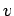. The instantaneous position of the object is most conveniently specified in terms of an angle. See Fig. 57. For instance, we could decide that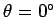corresponds to the object's location at, in which case we would write(245)

whereis termed the angular velocity of the object. For a uniformly rotating object, the angular velocity is simply the angle through which the object turns in one second.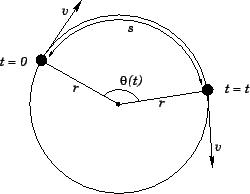Consider the motion of the object in the time interval betweenand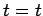. In this interval, the object rotates through an angle, and traces out a circular arc of length. See Fig. 57. It is fairly obvious that the arc lengthis directly proportional to the angle: but, what is the constant of proportionality? Well, an angle ofcorresponds to an arc length of. Hence, an anglemust correspond to an arc length of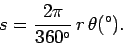(246)

At this stage, it is convenient to define a new angular unit known as a radian (symbol rad.). An angle measured in radians is related to an angle measured in degrees via the following simple formula:(247)

Thus,corresponds toradians,corresponds to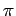radians,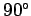corresponds to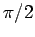radians, and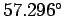corresponds to 1 radian. Whenis measured in radians, Eq. (246) simplifies greatly to give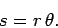(248)

Henceforth, in this course, all angles are measured in radians by default.

Consider the motion of the object in the short interval between timesand. In this interval, the object turns through a small angleand traces out a short arc of length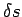, where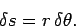(249)

Now(i.e., distance moved per unit time) is simply the tangential velocity, whereas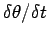(i.e., angle turned through per unit time) is simply the angular velocity. Thus, dividing Eq. (249) by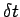, we obtain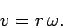(250)

Note, however, that this formula is only valid if the angular velocityis measured in radians per second. From now on, in this course, all angular velocities are measured in radians per second by default.

An object that rotates with uniform angular velocityturns throughradians in 1 second. Hence, the object turns throughradians (i.e., it executes a complete circle) in(251)

seconds. Here,is the repetition period of the circular motion. If the object executes a complete cycle (i.e., turns through) inseconds, then the number of cycles executed per second is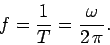(252)

Here, the repetition frequency,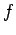, of the motion is measured in cycles per second--otherwise known as hertz (symbol Hz).

As an example, suppose that an object executes uniform circular motion, radius, at a frequency of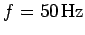(i.e., the object executes a complete rotation 50 times a second). The repetition period of this motion is simply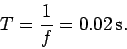(253)

Furthermore, the angular frequency of the motion is given by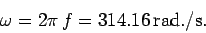(254)

Finally, the tangential velocity of the object is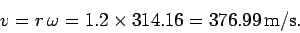(255)Next: Centripetal acceleration Up: Circular motion Previous: Introduction
Richard Fitzpatrick 2006-02-02